x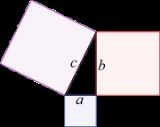Pythagorean theoremOverview

In mathematics
Mathematics
Mathematics is the study of quantity, space, structure, and change. Mathematicians seek out patterns and formulate new conjectures. Mathematicians resolve the truth or falsity of conjectures by mathematical proofs, which are arguments sufficient to convince other mathematicians of their validity...

, the Pythagorean theorem or Pythagoras' theorem is a relation in Euclidean geometry
Euclidean geometry
Euclidean geometry is a mathematical system attributed to the Alexandrian Greek mathematician Euclid, which he described in his textbook on geometry: the Elements. Euclid's method consists in assuming a small set of intuitively appealing axioms, and deducing many other propositions from these...

among the three sides of a right triangle
Right triangle
A right triangle or right-angled triangle is a triangle in which one angle is a right angle . The relation between the sides and angles of a right triangle is the basis for trigonometry.-Terminology:The side opposite the right angle is called the hypotenuse...

(right-angled triangle). In terms of areas, it states:

In any right angled triangle, the area of the square whose side is the hypotenuse
Hypotenuse
In geometry, a hypotenuse is the longest side of a right-angled triangle, the side opposite the right angle. The length of the hypotenuse of a right triangle can be found using the Pythagorean theorem, which states that the square of the length of the hypotenuse equals the sum of the squares of the...

(the side opposite the right angle) is equal to the sum of the areas of the squares whose sides are the two legs (the two sides that meet at a right angle
Right angle
In geometry and trigonometry, a right angle is an angle that bisects the angle formed by two halves of a straight line. More precisely, if a ray is placed so that its endpoint is on a line and the adjacent angles are equal, then they are right angles...

).

The theorem
Theorem
In mathematics, a theorem is a statement that has been proven on the basis of previously established statements, such as other theorems, and previously accepted statements, such as axioms...

can be written as an equation
Equation
An equation is a mathematical statement that asserts the equality of two expressions. In modern notation, this is written by placing the expressions on either side of an equals sign , for examplex + 3 = 5\,asserts that x+3 is equal to 5...

relating the lengths of the sides a, b and c, often called the Pythagorean equation: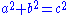where c represents the length of the hypotenuse, and a and b represent the lengths of the other two sides.

The Pythagorean theorem is named after the Greek mathematician Pythagoras
Pythagoras
Pythagoras of Samos was an Ionian Greek philosopher, mathematician, and founder of the religious movement called Pythagoreanism. Most of the information about Pythagoras was written down centuries after he lived, so very little reliable information is known about him...

, who by tradition is credited with its discovery and proof
Mathematical proof
In mathematics, a proof is a convincing demonstration that some mathematical statement is necessarily true. Proofs are obtained from deductive reasoning, rather than from inductive or empirical arguments. That is, a proof must demonstrate that a statement is true in all cases, without a single...

, although it is often argued that knowledge of the theorem predates him.How do you get the answer after you get almost done after you solve a,bWhat is the history of the pythagorean theoremDiscussionsPythagrean theromhow many forms of pythagorean theorem are there and what are they?pythagorean theoremEncyclopedia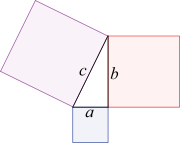In mathematics
Mathematics
Mathematics is the study of quantity, space, structure, and change. Mathematicians seek out patterns and formulate new conjectures. Mathematicians resolve the truth or falsity of conjectures by mathematical proofs, which are arguments sufficient to convince other mathematicians of their validity...

, the Pythagorean theorem or Pythagoras' theorem is a relation in Euclidean geometry
Euclidean geometry
Euclidean geometry is a mathematical system attributed to the Alexandrian Greek mathematician Euclid, which he described in his textbook on geometry: the Elements. Euclid's method consists in assuming a small set of intuitively appealing axioms, and deducing many other propositions from these...

among the three sides of a right triangle
Right triangle
A right triangle or right-angled triangle is a triangle in which one angle is a right angle . The relation between the sides and angles of a right triangle is the basis for trigonometry.-Terminology:The side opposite the right angle is called the hypotenuse...

(right-angled triangle). In terms of areas, it states:

In any right angled triangle, the area of the square whose side is the hypotenuse
Hypotenuse
In geometry, a hypotenuse is the longest side of a right-angled triangle, the side opposite the right angle. The length of the hypotenuse of a right triangle can be found using the Pythagorean theorem, which states that the square of the length of the hypotenuse equals the sum of the squares of the...

(the side opposite the right angle) is equal to the sum of the areas of the squares whose sides are the two legs (the two sides that meet at a right angle
Right angle
In geometry and trigonometry, a right angle is an angle that bisects the angle formed by two halves of a straight line. More precisely, if a ray is placed so that its endpoint is on a line and the adjacent angles are equal, then they are right angles...

).

The theorem
Theorem
In mathematics, a theorem is a statement that has been proven on the basis of previously established statements, such as other theorems, and previously accepted statements, such as axioms...

can be written as an equation
Equation
An equation is a mathematical statement that asserts the equality of two expressions. In modern notation, this is written by placing the expressions on either side of an equals sign , for examplex + 3 = 5\,asserts that x+3 is equal to 5...

relating the lengths of the sides a, b and c, often called the Pythagorean equation:where c represents the length of the hypotenuse, and a and b represent the lengths of the other two sides.

The Pythagorean theorem is named after the Greek mathematician Pythagoras
Pythagoras
Pythagoras of Samos was an Ionian Greek philosopher, mathematician, and founder of the religious movement called Pythagoreanism. Most of the information about Pythagoras was written down centuries after he lived, so very little reliable information is known about him...

, who by tradition is credited with its discovery and proof
Mathematical proof
In mathematics, a proof is a convincing demonstration that some mathematical statement is necessarily true. Proofs are obtained from deductive reasoning, rather than from inductive or empirical arguments. That is, a proof must demonstrate that a statement is true in all cases, without a single...

, although it is often argued that knowledge of the theorem predates him. There is evidence that Babylonian mathematicians
Babylonian mathematics
Babylonian mathematics refers to any mathematics of the people of Mesopotamia, from the days of the early Sumerians to the fall of Babylon in 539 BC. Babylonian mathematical texts are plentiful and well edited...

understood the formula, although there is little surviving evidence that they fitted it into a mathematical framework.

The theorem has numerous proof
Mathematical proof
In mathematics, a proof is a convincing demonstration that some mathematical statement is necessarily true. Proofs are obtained from deductive reasoning, rather than from inductive or empirical arguments. That is, a proof must demonstrate that a statement is true in all cases, without a single...

s, possibly the most of any mathematical theorem. These are very diverse, including both geometric proofs and algebraic proofs, with some dating back thousands of years. The theorem can be generalized in various ways, including higher-dimensional spaces, to spaces that are not Euclidean, to objects that are not right triangles, and indeed, to objects that are not triangles at all, but n-dimensional solids. The Pythagorean theorem has attracted interest outside mathematics as a symbol of mathematical abstruseness, mystique, or intellectual power; popular references in literature, plays, musicals, songs, stamps and cartoons abound.

## Other forms

As pointed out in the introduction, if c denotes the length
Length
In geometric measurements, length most commonly refers to the longest dimension of an object.In certain contexts, the term "length" is reserved for a certain dimension of an object along which the length is measured. For example it is possible to cut a length of a wire which is shorter than wire...

of the hypotenuse and a and b denote the lengths of the other two sides, the Pythagorean theorem can be expressed as the Pythagorean equation:If the length of both a and b are known, then c can be calculated as follows: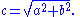If the length of hypotenuse c and one leg (a or b) are known, then the length of the other leg can be calculated with the following equations:orThe Pythagorean equation relates the sides of a right triangle in a simple way, so that if the lengths of any two sides are known the length of the third side can be found. Another corollary of the theorem is that in any right triangle, the hypotenuse is greater than any one of the legs, but less than the sum of them.

A generalization of this theorem is the law of cosines
Law of cosines
In trigonometry, the law of cosines relates the lengths of the sides of a plane triangle to the cosine of one of its angles. Using notation as in Fig...

, which allows the computation of the length of the third side of any triangle, given the lengths of two sides and the size of the angle between them. If the angle between the sides is a right angle, the law of cosines reduces to the Pythagorean equation.

## Proofs

This theorem may have more known proofs than any other (the law of quadratic reciprocity
In number theory, the law of quadratic reciprocity is a theorem about modular arithmetic which gives conditions for the solvability of quadratic equations modulo prime numbers...

being another contender for that distinction); the book The Pythagorean Proposition contains 370 proofs.

### Proof using similar triangles

This proof is based on the proportionality
Proportionality (mathematics)
In mathematics, two variable quantities are proportional if one of them is always the product of the other and a constant quantity, called the coefficient of proportionality or proportionality constant. In other words, are proportional if the ratio \tfrac yx is constant. We also say that one...

of the sides of two similar
Similarity (geometry)
Two geometrical objects are called similar if they both have the same shape. More precisely, either one is congruent to the result of a uniform scaling of the other...

triangles, that is, upon the fact that the ratio
Ratio
In mathematics, a ratio is a relationship between two numbers of the same kind , usually expressed as "a to b" or a:b, sometimes expressed arithmetically as a dimensionless quotient of the two which explicitly indicates how many times the first number contains the second In mathematics, a ratio is...

of any two corresponding sides of similar triangles is the same regardless of the size of the triangles.

Let ABC represent a right triangle, with the right angle located at C, as shown on the figure. We draw the altitude
Altitude (triangle)
In geometry, an altitude of a triangle is a straight line through a vertex and perpendicular to a line containing the base . This line containing the opposite side is called the extended base of the altitude. The intersection between the extended base and the altitude is called the foot of the...

from point C, and call H its intersection with the side AB. Point H divides the length of the hypotenuse c into parts d and e. The new triangle ACH is similar
Similarity (geometry)
Two geometrical objects are called similar if they both have the same shape. More precisely, either one is congruent to the result of a uniform scaling of the other...

to triangle ABC, because they both have a right angle (by definition of the altitude), and they share the angle at A, meaning that the third angle will be the same in both triangles as well, marked as θ in the figure. By a similar reasoning, the triangle CBH is also similar to ABC. The proof of similarity of the triangles requires the Triangle postulate
Triangle postulate
In Euclidean geometry, the triangle postulate states that the sum of the angles of a triangle is two right angles. This postulate is equivalent to the parallel postulate...

: the sum of the angles in a triangle is two right angles, and is equivalent to the parallel postulate
Parallel postulate
In geometry, the parallel postulate, also called Euclid's fifth postulate because it is the fifth postulate in Euclid's Elements, is a distinctive axiom in Euclidean geometry...

. Similarity of the triangles leads to the equality of ratios of corresponding sides: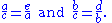The first result equates the cosine of each angle θ and the second result equates the sine
Sine
In mathematics, the sine function is a function of an angle. In a right triangle, sine gives the ratio of the length of the side opposite to an angle to the length of the hypotenuse.Sine is usually listed first amongst the trigonometric functions....

s.

These ratios can be written as: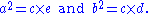Summing these two equalities, we obtainwhich, tidying up, is the Pythagorean theorem: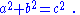The role of this proof in history is the subject of much speculation. The underlying question is why Euclid did not use this proof, but invented another. One conjecture is that the proof by similar triangles involved a theory of proportions, a topic not discussed until later in the Elements, and that the theory of proportions needed further development at that time.

### Euclid's proof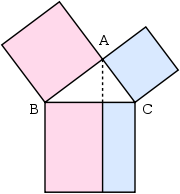In outline, here is how the proof in Euclid's
Euclid
Euclid , fl. 300 BC, also known as Euclid of Alexandria, was a Greek mathematician, often referred to as the "Father of Geometry". He was active in Alexandria during the reign of Ptolemy I...

Elements
Euclid's Elements
Euclid's Elements is a mathematical and geometric treatise consisting of 13 books written by the Greek mathematician Euclid in Alexandria c. 300 BC. It is a collection of definitions, postulates , propositions , and mathematical proofs of the propositions...

proceeds. The large square is divided into a left and right rectangle. A triangle is constructed that has half the area of the left rectangle. Then another triangle is constructed that has half the area of the square on the left-most side. These two triangles are shown to be congruent, proving this square has the same area as the left rectangle. This argument is followed by a similar version for the right rectangle and the remaining square. Putting the two rectangles together to reform the square on the hypotenuse, its area is the same as the sum of the area of the other two squares. The details are next.

Let A, B, C be the vertices
Vertex (geometry)
In geometry, a vertex is a special kind of point that describes the corners or intersections of geometric shapes.-Of an angle:...

of a right triangle, with a right angle at A. Drop a perpendicular from A to the side opposite the hypotenuse in the square on the hypotenuse. That line divides the square on the hypotenuse into two rectangles, each having the same area as one of the two squares on the legs.

For the formal proof, we require four elementary lemmata
Lemma (mathematics)
In mathematics, a lemma is a proven proposition which is used as a stepping stone to a larger result rather than as a statement in-and-of itself...

:
1. If two triangles have two sides of the one equal to two sides of the other, each to each, and the angles included by those sides equal, then the triangles are congruent (side-angle-side).
2. The area of a triangle is half the area of any parallelogram on the same base and having the same altitude.
3. The area of a rectangle is equal to the product of two adjacent sides.
4. The area of a square is equal to the product of two of its sides (follows from 3).

Next, each top square is related to a triangle congruent with another triangle related in turn to one of two rectangles making up the lower square.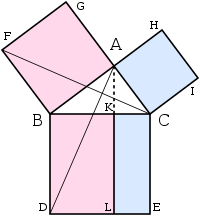The proof is as follows:
1. Let ACB be a right-angled triangle with right angle CAB.
2. On each of the sides BC, AB, and CA, squares are drawn, CBDE, BAGF, and ACIH, in that order. The construction of squares requires the immediately preceding theorems in Euclid, and depends upon the parallel postulate.
3. From A, draw a line parallel to BD and CE. It will perpendicularly intersect BC and DE at K and L, respectively.
4. Join CF and AD, to form the triangles BCF and BDA.
5. Angles CAB and BAG are both right angles; therefore C, A, and G are collinear. Similarly for B, A, and H.
6. Angles CBD and FBA are both right angles; therefore angle ABD equals angle FBC, since both are the sum of a right angle and angle ABC.
7. Since AB is equal to FB and BD is equal to BC, triangle ABD must be congruent to triangle FBC.
8. Since A-K-L is a straight line, parallel to BD, then parallelogram BDLK has twice the area of triangle ABD because they share the base BD and have the same altitude BK, i.e., a line normal to their common base, connecting the parallel lines BD and AL. (lemma 2)
9. Since C is collinear with A and G, square BAGF must be twice in area to triangle FBC.
10. Therefore rectangle BDLK must have the same area as square BAGF = AB2.
11. Similarly, it can be shown that rectangle CKLE must have the same area as square ACIH = AC2.
12. Adding these two results, AB2 + AC2 = BD × BK + KL × KC
13. Since BD = KL, BD × BK + KL × KC = BD(BK + KC) = BD × BC
14. Therefore AB2 + AC2 = BC2, since CBDE is a square.

This proof, which appears in Euclid's Elements as that of Proposition 47 in Book 1, demonstrates that the area of the square on the hypotenuse is the sum of the areas of the other two squares. This is quite distinct from the proof by similarity of triangles, which is conjectured to be the proof that Pythagoras used.

### Proof by rearrangement

The leftmost animation consists of a large square, side , containing four right triangles. The triangles are shown in two arrangements. The first of which leaves a square c2 uncovered, the second leaving two squares a2 and b2 uncovered. But as these with the triangles fill the large square they must be equal, so

A second proof is given by the middle animation. A large square is formed with area c2, from four identical right triangles with sides a, b and c, fitted around a small central square. Then two rectangles are formed with sides a and b by moving the triangles. Combining the smaller square with these rectangles produces two squares of areas a2 and b2, which must have the same area as the initial large square.

The third, rightmost image also gives a proof. The upper two squares are divided as shown by the blue and green shading, into pieces that when rearranged can be made to fit in the lower square on the hypotenuse – or conversely the large square can be divided as shown into pieces that fill the other two. This shows the area of the large square equals that of the two smaller ones.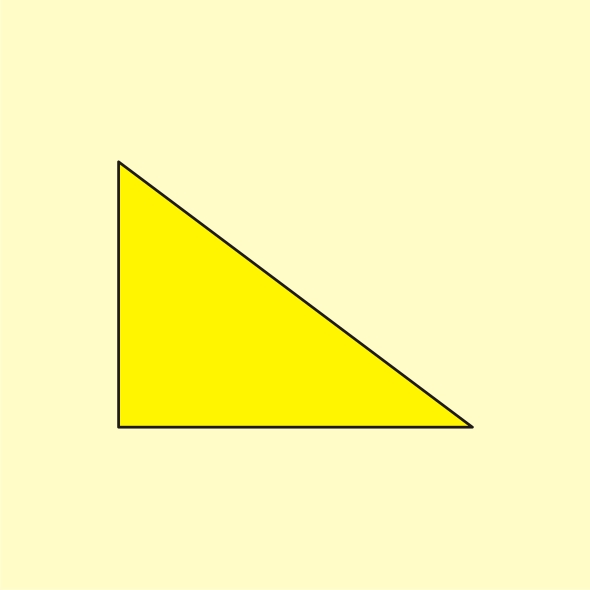### Algebraic proofs

The theorem can be proved algebraically using four copies of a right triangle with sides a, b and c, arranged inside a square with side c as in the top half of the diagram. The triangles are similar with area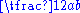, while the small square has side and area . The area of the large square is therefore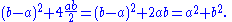But this is a square with side c and area c2, so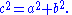A similar proof uses four copies of the same triangle arranged symmetrically around a square with side c, as shown in the lower part of the diagram. This results in a larger square, with side and area . The four triangles and the square side c must have the same area as the larger square,givingA related proof was published by U.S. President James A. Garfield. Instead of a square it uses a trapezoid
Trapezoid
In Euclidean geometry, a convex quadrilateral with one pair of parallel sides is referred to as a trapezoid in American English and as a trapezium in English outside North America. A trapezoid with vertices ABCD is denoted...

, which can be constructed from the square in the second of the above proofs by bisecting along a diagonal of the inner square, to give the trapezoid as shown in the diagram. The area of the trapezoid can be calculated to be half the area of the square, that isThe inner square is similarly halved, and there are only two triangles so the proof proceeds as above except for a factor of, which is removed by multiplying by two to give the result.

### Proof using differentials

One can arrive at the Pythagorean theorem by studying how changes in a side produce a change in the hypotenuse and employing calculus
Calculus
Calculus is a branch of mathematics focused on limits, functions, derivatives, integrals, and infinite series. This subject constitutes a major part of modern mathematics education. It has two major branches, differential calculus and integral calculus, which are related by the fundamental theorem...

.

The triangle ABC is a right triangle, as shown in the upper part of the diagram, with BC the hypotenuse. At the same time the triangle lengths are measured as shown, with the hypotenuse of length y, the side AC of length x and the side AB of length a, as seen in the lower diagram part.
If x is increased by a small amount dx by extending the side AC slightly to D, then y also increases by dy. These form two sides of a triangle, CDE, which (with E chosen so CE is perpendicular to the hypotenuse) is a right triangle approximately similar to ABC. Therefore the ratios of their sides must be the same, that is: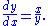This can be rewritten as follows: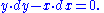This is a differential equation
Differential equation
A differential equation is a mathematical equation for an unknown function of one or several variables that relates the values of the function itself and its derivatives of various orders...

which is solved to give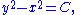And the constant can be deduced from x = 0, y = a to give the equationThis is more of an intuitive proof than a formal one: it can be made more rigorous if proper limits are used in place of dx and dy.

## Converse

The converse of the theorem is also true:
For any three positive numbers a, b, and c such that , there exists a triangle with sides a, b and c, and every such triangle has a right angle between the sides of lengths a and b.

An alternative statement is:

For any triangle with sides a, b, c, if then the angle between a and b measures 90°.

This converse also appears in Euclid's Elements (Book I, Proposition 48):

It can be proven using the law of cosines
Law of cosines
In trigonometry, the law of cosines relates the lengths of the sides of a plane triangle to the cosine of one of its angles. Using notation as in Fig...

or as follows:

Let ABC be a triangle with side lengths a, b, and c, with Construct a second triangle with sides of length a and b containing a right angle. By the Pythagorean theorem, it follows that the hypotenuse of this triangle has length c = , the same as the hypotenuse of the first triangle. Since both triangles' sides are the same lengths a, b and c, the triangles are congruent
Congruence (geometry)
In geometry, two figures are congruent if they have the same shape and size. This means that either object can be repositioned so as to coincide precisely with the other object...

and must have the same angles. Therefore, the angle between the side of lengths a and b in the original triangle is a right angle.

The above proof of the converse makes use of the Pythagorean Theorem itself. The converse can also be proven without assuming the Pythagorean Theorem.

A corollary
Corollary
A corollary is a statement that follows readily from a previous statement.In mathematics a corollary typically follows a theorem. The use of the term corollary, rather than proposition or theorem, is intrinsically subjective...

of the Pythagorean theorem's converse is a simple means of determining whether a triangle is right, obtuse, or acute, as follows. Let c be chosen to be the longest of the three sides and (otherwise there is no triangle according to the triangle inequality
Triangle inequality
In mathematics, the triangle inequality states that for any triangle, the sum of the lengths of any two sides must be greater than or equal to the length of the remaining side ....

). The following statements apply:
• If then the triangle is right.
• If then the triangle is acute.
• If then the triangle is obtuse.

Edsger Dijkstra
Edsger Dijkstra
Edsger Wybe Dijkstra ; ) was a Dutch computer scientist. He received the 1972 Turing Award for fundamental contributions to developing programming languages, and was the Schlumberger Centennial Chair of Computer Sciences at The University of Texas at Austin from 1984 until 2000.Shortly before his...

has stated this proposition about acute, right, and obtuse triangles in this language:

where α is the angle opposite to side a, β is the angle opposite to side b, γ is the angle opposite to side c, and sgn is the sign function
Sign function
In mathematics, the sign function is an odd mathematical function that extracts the sign of a real number. To avoid confusion with the sine function, this function is often called the signum function ....

.

### Pythagorean triples

A Pythagorean triple has three positive integers a, b, and c, such that In other words, a Pythagorean triple represents the lengths of the sides of a right triangle where all three sides have integer lengths. Evidence from megalithic monuments in Northern Europe shows that such triples were known before the discovery of writing. Such a triple is commonly written Some well-known examples are and

A primitive Pythagorean triple is one in which a, b and c are coprime
Coprime
In number theory, a branch of mathematics, two integers a and b are said to be coprime or relatively prime if the only positive integer that evenly divides both of them is 1. This is the same thing as their greatest common divisor being 1...

(the greatest common divisor
Greatest common divisor
In mathematics, the greatest common divisor , also known as the greatest common factor , or highest common factor , of two or more non-zero integers, is the largest positive integer that divides the numbers without a remainder.For example, the GCD of 8 and 12 is 4.This notion can be extended to...

of a, b and c is 1).

The following is a list of primitive Pythagorean triples with values less than 100:

, (5, 12, 13), (7, 24, 25), (8, 15, 17), (9, 40, 41), (11, 60, 61), (12, 35, 37), (13, 84, 85), (16, 63, 65), (20, 21, 29), (28, 45, 53), (33, 56, 65), (36, 77, 85), (39, 80, 89), (48, 55, 73), (65, 72, 97)

### Incommensurable lengths

One of the consequences of the Pythagorean theorem is that line segments whose lengths are incommensurable
Commensurability (mathematics)
In mathematics, two non-zero real numbers a and b are said to be commensurable if a/b is a rational number.-History of the concept:...

(so the ratio of which is not a rational number
Rational number
In mathematics, a rational number is any number that can be expressed as the quotient or fraction a/b of two integers, with the denominator b not equal to zero. Since b may be equal to 1, every integer is a rational number...

) can be constructed using a straightedge and compass. Pythagoras' theorem enables construction of incommensurable lengths because the hypotenuse of a triangle is related to the sides by the square root
Square root
In mathematics, a square root of a number x is a number r such that r2 = x, or, in other words, a number r whose square is x...

operation.

The figure on the right shows how to construct line segments whose lengths are in the ratio of the square root of any positive integer. Each triangle has a side (labeled "1") that is the chosen unit for measurement. In each right triangle, Pythagoras' theorem establishes the length of the hypotenuse in terms of this unit. If a hypotenuse is related to the unit by the square root of a positive integer that is not a perfect square, it is a realization of a length incommensurable with the unit, such as , ,  . For more detail, see Quadratic irrational
In mathematics, a quadratic irrational is an irrational number that is the solution to some quadratic equation with rational coefficients...

.

Incommensurable lengths conflicted with the Pythagorean school's concept of numbers as only whole numbers. The Pythagorean school dealt with proportions by comparison of integer multiples of a common subunit. According to one legend, Hippasus of Metapontum
Hippasus
Hippasus of Metapontum in Magna Graecia, was a Pythagorean philosopher. Little is known about his life or his beliefs, but he is sometimes credited with the discovery of the existence of irrational numbers.-Life:...

(ca. 470 B.C.) was drowned at sea for making known the existence of the irrational or incommensurable.

### Complex numbersFor any complex number
Complex number
A complex number is a number consisting of a real part and an imaginary part. Complex numbers extend the idea of the one-dimensional number line to the two-dimensional complex plane by using the number line for the real part and adding a vertical axis to plot the imaginary part...the absolute value
Absolute value
In mathematics, the absolute value |a| of a real number a is the numerical value of a without regard to its sign. So, for example, the absolute value of 3 is 3, and the absolute value of -3 is also 3...

or modulus is given by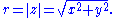So the three quantities, r, x and y are related by the Pythagorean equation,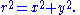Note that r is defined to be a positive number or zero but x and y can be negative as well as positive. Geometrically r is the distance of the z from zero or the origin O in the complex plane
Complex plane
In mathematics, the complex plane or z-plane is a geometric representation of the complex numbers established by the real axis and the orthogonal imaginary axis...

.

This can be generalised to find the distance between two points, z1 and z2 say. The required distance is given byso again they are related by a version of the Pythagorean equation,### Euclidean distance in various coordinate systems

The distance formula in Cartesian coordinates is derived from the Pythagorean theorem. If and are points in the plane, then the distance between them, also called the Euclidean distance
Euclidean distance
In mathematics, the Euclidean distance or Euclidean metric is the "ordinary" distance between two points that one would measure with a ruler, and is given by the Pythagorean formula. By using this formula as distance, Euclidean space becomes a metric space...

, is given by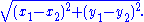More generally, in Euclidean n-space
Euclidean space
In mathematics, Euclidean space is the Euclidean plane and three-dimensional space of Euclidean geometry, as well as the generalizations of these notions to higher dimensions...

, the Euclidean distance between two points,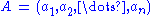and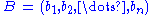, is defined, by generalization of the Pythagorean theorem, as:If Cartesian coordinates are not used, for example, if polar coordinates are used in two dimensions or, in more general terms, if curvilinear coordinates
Curvilinear coordinates
Curvilinear coordinates are a coordinate system for Euclidean space in which the coordinate lines may be curved. These coordinates may be derived from a set of Cartesian coordinates by using a transformation that is locally invertible at each point. This means that one can convert a point given...

are used, the formulas expressing the Euclidean distance are more complicated than the Pythagorean theorem, but can be derived from it. A typical example where the straight-line distance between two points is converted to curvilinear coordinates can be found in the applications of Legendre polynomials in physics. The formulas can be discovered by using Pythagoras' theorem with the equations relating the curvilinear coordinates to Cartesian coordinates. For example, the polar coordinates can be introduced as:Then two points with locations and are separated by a distance s: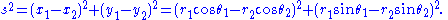Performing the squares and combining terms, the Pythagorean formula for distance in Cartesian coordinates produces the separation in polar coordinates as:using the trigonometric product-to-sum formulas. This formula is the law of cosines, sometimes called the Generalized Pythagorean Theorem. From this result, for the case where the radii to the two locations are at right angles, the enclosed angle and the form corresponding to Pythagoras' theorem is regained: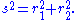The Pythagorean theorem, valid for right triangles, therefore is a special case of the more general law of cosines, valid for arbitrary triangles.

### Pythagorean trigonometric identity

In a right triangle with sides a, b and hypotenuse c, trigonometry
Trigonometry
Trigonometry is a branch of mathematics that studies triangles and the relationships between their sides and the angles between these sides. Trigonometry defines the trigonometric functions, which describe those relationships and have applicability to cyclical phenomena, such as waves...

determines the sine
Sine
In mathematics, the sine function is a function of an angle. In a right triangle, sine gives the ratio of the length of the side opposite to an angle to the length of the hypotenuse.Sine is usually listed first amongst the trigonometric functions....

and cosine of the angle θ between side a and the hypotenuse as:From that it follows:where the last step applies Pythagoras' theorem. This relation between sine and cosine sometimes is called the fundamental Pythagorean trigonometric identity. In similar triangles, the ratios of the sides are the same regardless of the size of the triangles, and depend upon the angles. Consequently, in the figure, the triangle with hypotenuse of unit size has opposite side of size sin θ and adjacent side of size cos θ in units of the hypotenuse.

### Relation to the cross product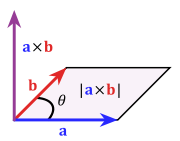The Pythagorean theorem relates the cross product
Cross product
In mathematics, the cross product, vector product, or Gibbs vector product is a binary operation on two vectors in three-dimensional space. It results in a vector which is perpendicular to both of the vectors being multiplied and normal to the plane containing them...

and dot product
Dot product
In mathematics, the dot product or scalar product is an algebraic operation that takes two equal-length sequences of numbers and returns a single number obtained by multiplying corresponding entries and then summing those products...

in a similar way: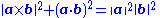This can be seen from the definitions of the cross product and dot product, as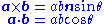with n a unit vector normal to both a and b. The relationship follows from these definitions and the Pythagorean trigonometric identity.

This can also be used to define the cross product. By rearranging the following equation is obtainedThis can be considered as a condition on the cross product and so part of its definition, for example in seven dimensions.

### Similar figures on the three sides

The Pythagorean theorem was generalized by Euclid
Euclid
Euclid , fl. 300 BC, also known as Euclid of Alexandria, was a Greek mathematician, often referred to as the "Father of Geometry". He was active in Alexandria during the reign of Ptolemy I...

in his Elements
Euclid's Elements
Euclid's Elements is a mathematical and geometric treatise consisting of 13 books written by the Greek mathematician Euclid in Alexandria c. 300 BC. It is a collection of definitions, postulates , propositions , and mathematical proofs of the propositions...

to extend beyond the areas of squares on the three sides to similar figures:

If one erects similar figures (see Euclidean geometry
Euclidean geometry
Euclidean geometry is a mathematical system attributed to the Alexandrian Greek mathematician Euclid, which he described in his textbook on geometry: the Elements. Euclid's method consists in assuming a small set of intuitively appealing axioms, and deducing many other propositions from these...

) on the sides of a right triangle, then the sum of the areas of the two smaller ones equals the area of the larger one.

The basic idea behind this generalization is that the area of a plane figure is proportional
Proportionality (mathematics)
In mathematics, two variable quantities are proportional if one of them is always the product of the other and a constant quantity, called the coefficient of proportionality or proportionality constant. In other words, are proportional if the ratio \tfrac yx is constant. We also say that one...

to the square of any linear dimension, and in particular is proportional to the square of the length of any side. Thus, if similar figures with areas A, B and C are erected on sides with lengths a, b and c then: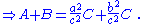But, by the Pythagorean theorem, a2 + b2 = c2, so A + B = C.

Conversely, if we can prove that A + B = C for three similar figures without using the Pythagorean theorem, then we can work backwards to construct a proof of the theorem. For example, the starting center triangle can be replicated and used as a triangle C on its hypotenuse, and two similar right triangles (A and B ) constructed on the other two sides, formed by dividing the central triangle by its altitude
Altitude (triangle)
In geometry, an altitude of a triangle is a straight line through a vertex and perpendicular to a line containing the base . This line containing the opposite side is called the extended base of the altitude. The intersection between the extended base and the altitude is called the foot of the...

. The sum of the areas of the two smaller triangles therefore is that of the third, thus A + B = C and reversing the above logic leads to the Pythagorean theorem a2 + b2 = c2.

### Law of cosines

The Pythagorean theorem is a special case of the more general theorem relating the lengths of sides in any triangle, the law of cosines:where θ is the angle between sides a and b.

When θ is 90 degrees, then cosθ = 0, and the formula reduces to the usual Pythagorean theorem.

### Arbitrary triangle

At any selected angle of a general triangle of sides a, b, c, inscribe an isosceles triangle such that the equal angles at its base θ are the same as the selected angle. Suppose the selected angle θ is opposite the side labeled c. Inscribing the isosceles triangle forms triangle ABD with angle θ opposite side a and with side r along c. A second triangle is formed with angle θ opposite side b and a side with length s along c, as shown in the figure. Tâbit ibn Qorra
Thabit ibn Qurra
' was a mathematician, physician, astronomer and translator of the Islamic Golden Age.Ibn Qurra made important discoveries in algebra, geometry and astronomy...

stated that the sides of the three triangles were related as: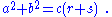As the angle θ approaches π/2, the base of the isosceles triangle narrows, and lengths r and s overlap less and less. When θ = π/2, ADB becomes a right triangle, r + s = c, and the original Pythagoras' theorem is regained.

One proof observes that triangle ABC has the same angles as triangle ABD, but in opposite order. (The two triangles share the angle at vertex B, both contain the angle θ, and so also have the same third angle by the triangle postulate
Triangle postulate
In Euclidean geometry, the triangle postulate states that the sum of the angles of a triangle is two right angles. This postulate is equivalent to the parallel postulate...

.) Consequently, ABC is similar to the reflection of ABD, the triangle DBA in the lower panel. Taking the ratio of sides opposite and adjacent to θ,Likewise, for the reflection of the other triangle,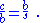Clearing fractions and adding these two relations:the required result.

### General triangles using parallelograms

A further generalization applies to triangles that are not right triangles, using parallelograms on the three sides in place of squares. (Squares are a special case, of course.) The upper figure shows that for a scalene triangle, the area of the parallelogram on the longest side is the sum of the areas of the parallelograms on the other two sides, provided the parallelogram on the long side is constructed as indicated (the dimensions labeled with arrows are the same, and determine the sides of the bottom parallelogram). This replacement of squares with parallelograms bears a clear resemblance to the original Pythagoras' theorem, and was considered a generalization by Pappus of Alexandria
Pappus of Alexandria
Pappus of Alexandria was one of the last great Greek mathematicians of Antiquity, known for his Synagoge or Collection , and for Pappus's Theorem in projective geometry...

in 4 A.D.

The lower figure shows the elements of the proof. Focus on the left side of the figure. The left green parallelogram has the same area as the left, blue portion of the bottom parallelogram because both have the same base b and height h. However, the left green parallelogram also has the same area as the left green parallelogram of the upper figure, because they have the same base (the upper left side of the triangle) and the same height normal to that side of the triangle. Repeating the argument for the right side of the figure, the bottom parallelogram has the same area as the sum of the two green parallelograms.

### Solid geometryIn terms of solid geometry, Pythagoras' theorem can be applied to three dimensions as follows. Consider a rectangular solid as shown in the figure. The length of diagonal BD is found from Pythagoras' theorem as:where these three sides form a right triangle. Using horizontal diagonal BD and the vertical edge AB, the length of diagonal AD then is found by a second application of Pythagoras' theorem as:or, doing it all in one step: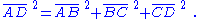This result is the three-dimensional expression for the magnitude of a vector v (the diagonal AD) in terms of its orthogonal components {vk} (the three mutually perpendicular sides):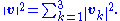This one-step formulation may be viewed as a generalization of Pythagoras' theorem to higher dimensions. However, this result is really just the repeated application of the original Pythagoras' theorem to a succession of right triangles in a sequence of orthogonal planes.

A substantial generalization of the Pythagorean theorem to three dimensions is de Gua's theorem, named for Jean Paul de Gua de Malves
Jean Paul de Gua de Malves
Jean Paul de Gua de Malves was a French mathematician who published in 1740 a work on analytical geometry in which he applied it, without the aid of differential calculus, to find the tangents, asymptotes, and various singular points of an algebraic curve.He further showed how singular points and...

: If a tetrahedron
Tetrahedron
In geometry, a tetrahedron is a polyhedron composed of four triangular faces, three of which meet at each vertex. A regular tetrahedron is one in which the four triangles are regular, or "equilateral", and is one of the Platonic solids...

has a right angle corner (a corner like a cube), then the square of the area of the face opposite the right angle corner is the sum of the squares of the areas of the other three faces. This result can be generalized as in the "n-dimensional Pythagorean theorem":
This statement is illustrated in three dimensions by the tetrahedron in the figure. The "hypotenuse" is the base of the tetrahedron at the back of the figure, and the "legs" are the three sides emanating from the vertex in the foreground. As the depth of the base from the vertex increases, the area of the "legs" increases, while that of the base is fixed. The theorem suggests that when this depth is at the value creating a right vertex, the generalization of Pythagoras' theorem applies. In a different wording:

### Inner product spaces

The Pythagorean theorem can be generalized to inner product space
Inner product space
In mathematics, an inner product space is a vector space with an additional structure called an inner product. This additional structure associates each pair of vectors in the space with a scalar quantity known as the inner product of the vectors...

s, which are generalizations of the familiar 2-dimensional and 3-dimensional Euclidean spaces. For example, a function
Function (mathematics)
In mathematics, a function associates one quantity, the argument of the function, also known as the input, with another quantity, the value of the function, also known as the output. A function assigns exactly one output to each input. The argument and the value may be real numbers, but they can...

may be considered as a vector
Vector space
A vector space is a mathematical structure formed by a collection of vectors: objects that may be added together and multiplied by numbers, called scalars in this context. Scalars are often taken to be real numbers, but one may also consider vector spaces with scalar multiplication by complex...

with infinitely many components in an inner product space, as in functional analysis
Functional analysis
Functional analysis is a branch of mathematical analysis, the core of which is formed by the study of vector spaces endowed with some kind of limit-related structure and the linear operators acting upon these spaces and respecting these structures in a suitable sense...

.

In an inner product space, the concept of perpendicular
Perpendicular
In geometry, two lines or planes are considered perpendicular to each other if they form congruent adjacent angles . The term may be used as a noun or adjective...

ity is replaced by the concept of orthogonality: two vectors v and w are orthogonal if their inner productis zero. The inner product is a generalization of the dot product
Dot product
In mathematics, the dot product or scalar product is an algebraic operation that takes two equal-length sequences of numbers and returns a single number obtained by multiplying corresponding entries and then summing those products...

of vectors. The dot product is called the standard inner product or the Euclidean inner product. However, other inner products are possible.

The concept of length is replaced by the concept of the norm
Normed vector space
In mathematics, with 2- or 3-dimensional vectors with real-valued entries, the idea of the "length" of a vector is intuitive and can easily be extended to any real vector space Rn. The following properties of "vector length" are crucial....

||v|| of a vector v, defined as: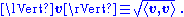In an inner-product space, the Pythagorean theorem states that for any two orthogonal vectors v and w we have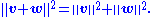Here the vectors v and w are akin to the sides of a right triangle with hypotenuse given by the vector sum v + w. This form of the Pythagorean theorem is a consequence of the properties of the inner product: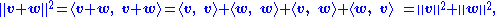where the inner products of the cross terms are zero, because of orthogonality.

A further generalization of the Pythagorean theorem in an inner product space to non-orthogonal vectors is the parallelogram law :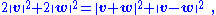which says that twice the sum of the squares of the lengths of the sides of a parallelogram is the sum of the squares of the lengths of the diagonals. Any norm that satisfies this equality is ipso facto
Ipso facto
Ipso facto is a Latin phrase, directly translated as "by the fact itself," which means that a certain phenomenon is a direct consequence, a resultant effect, of the action in question, instead of being brought about by a subsequent action such as the verdict of a tribunal. It is a term of art used...

a norm corresponding to an inner product.
The Pythagorean identity can be extended to sums of more than two orthogonal vectors. If v1, v2, ..., vn are pairwise-orthogonal vectors in an inner-product space, then application of the Pythagorean theorem to successive pairs of these vectors (as described for 3-dimensions in the section on solid geometry) results in the equation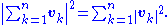Parseval's identity
Parseval's identity
In mathematical analysis, Parseval's identity is a fundamental result on the summability of the Fourier series of a function. Geometrically, it is thePythagorean theorem for inner-product spaces....

is a further generalization that considers infinite sums of orthogonal vectors.

For the inner product(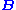is a Hermitian
Hermitian
A number of mathematical entities are named Hermitian, after the mathematician Charles Hermite:*Hermitian adjoint*Hermitian connection, the unique connection on a Hermitian manifold that satisfies specific conditions...

positive-definite matrix
Positive-definite matrix
In linear algebra, a positive-definite matrix is a matrix that in many ways is analogous to a positive real number. The notion is closely related to a positive-definite symmetric bilinear form ....

and u* the conjugate transpose
Conjugate transpose
In mathematics, the conjugate transpose, Hermitian transpose, Hermitian conjugate, or adjoint matrix of an m-by-n matrix A with complex entries is the n-by-m matrix A* obtained from A by taking the transpose and then taking the complex conjugate of each entry...

of u) the Pythagorean theorem is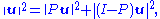where P is a projection
Projection (linear algebra)
In linear algebra and functional analysis, a projection is a linear transformation P from a vector space to itself such that P2 = P. It leaves its image unchanged....

which satisfies; the linear map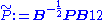then is an orthogonal projection.

### Non-Euclidean geometry

The Pythagorean theorem is derived from the axioms of Euclidean geometry
Euclidean geometry
Euclidean geometry is a mathematical system attributed to the Alexandrian Greek mathematician Euclid, which he described in his textbook on geometry: the Elements. Euclid's method consists in assuming a small set of intuitively appealing axioms, and deducing many other propositions from these...

, and in fact, the Pythagorean theorem given above does not hold in a non-Euclidean geometry
Non-Euclidean geometry
Non-Euclidean geometry is the term used to refer to two specific geometries which are, loosely speaking, obtained by negating the Euclidean parallel postulate, namely hyperbolic and elliptic geometry. This is one term which, for historical reasons, has a meaning in mathematics which is much...

. (The Pythagorean theorem has been shown, in fact, to be equivalent to Euclid's Parallel (Fifth) Postulate.)
In other words, in non-Euclidean geometry, the relation between the sides of a triangle must necessarily take a non-Pythagorean form. For example, in spherical geometry
Spherical geometry
Spherical geometry is the geometry of the two-dimensional surface of a sphere. It is an example of a geometry which is not Euclidean. Two practical applications of the principles of spherical geometry are to navigation and astronomy....

, all three sides of the right triangle (say a, b, and c) bounding an octant of the unit sphere have length equal to π/2, and all its angles are right angles, which violates the Pythagorean theorem because

Here two cases of non-Euclidean geometry are considered—spherical geometry
Spherical geometry
Spherical geometry is the geometry of the two-dimensional surface of a sphere. It is an example of a geometry which is not Euclidean. Two practical applications of the principles of spherical geometry are to navigation and astronomy....

and hyperbolic plane geometry
Hyperbolic geometry
In mathematics, hyperbolic geometry is a non-Euclidean geometry, meaning that the parallel postulate of Euclidean geometry is replaced...

; in each case, as in the Euclidean case for non-right triangles, the result replacing the Pythagorean theorem follows from the appropriate law of cosines.

However, the Pythagorean theorem remains true in hyperbolic geometry and elliptic geometry if the condition that the triangle be right is replaced with the condition that two of the angles sum to the third, say A+B = C. The sides are then related as follows: the sum of the areas of the circles with diameters a and b equals the area of the circle with diameter c.

#### Spherical geometry

For any right triangle on a sphere of radius R (for example, if γ in the figure is a right angle), with sides a, b, c, the relation between the sides takes the form: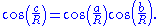This equation can be derived as a special case of the spherical law of cosines that applies to all spherical triangles:By using the Maclaurin series for the cosine function, , it can be shown that as the radius R approaches infinity and the arguments a/R, b/R and c/R tend to zero, the spherical relation between the sides of a right triangle approaches the form of Pythagoras' theorem. Substituting the approximate quadratic for each of the cosines in the spherical relation for a right triangle: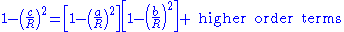Multiplying out the bracketed quantities, Pythagoras' theorem is recovered for large radii R: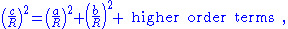where the higher order terms become negligibly small as R becomes large.

#### Hyperbolic geometryFor a right triangle in hyperbolic geometry with sides a, b, c and with side c opposite a right angle, the relation between the sides takes the form: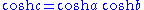where cosh is the hyperbolic cosine
Hyperbolic function
In mathematics, hyperbolic functions are analogs of the ordinary trigonometric, or circular, functions. The basic hyperbolic functions are the hyperbolic sine "sinh" , and the hyperbolic cosine "cosh" , from which are derived the hyperbolic tangent "tanh" and so on.Just as the points form a...

. This formula is a special form of the hyperbolic law of cosines that applies to all hyperbolic triangles: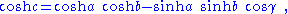with γ the angle at the vertex opposite the side c.

By using the Maclaurin series for the hyperbolic cosine, , it can be shown that as a hyperbolic triangle becomes very small (that is, as a, b, and c all approach zero), the hyperbolic relation for a right triangle approaches the form of Pythagoras' theorem.

### Differential geometry

On an infinitesimal level, in three dimensional space, Pythagoras' theorem describes the distance between two infinitesimally separated points as: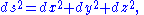with ds the element of distance and (dx, dy, dz) the components of the vector separating the two points. Such a space is called a Euclidean space
Euclidean space
In mathematics, Euclidean space is the Euclidean plane and three-dimensional space of Euclidean geometry, as well as the generalizations of these notions to higher dimensions...

. However, a generalization of this expression useful for general coordinates (not just Cartesian) and general spaces (not just Euclidean) takes the form: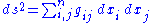where is called the metric tensor. It may be a function of position. Such curved space
Curved space
Curved space often refers to a spatial geometry which is not “flat” where a flat space is described by Euclidean geometry. Curved spaces can generally be described by Riemannian geometry though some simple cases can be described in other ways. Curved spaces play an essential role in General...

s include Riemannian geometry
Riemannian geometry
Riemannian geometry is the branch of differential geometry that studies Riemannian manifolds, smooth manifolds with a Riemannian metric, i.e. with an inner product on the tangent space at each point which varies smoothly from point to point. This gives, in particular, local notions of angle, length...

as a general example. This formulation also applies to a Euclidean space when using curvilinear coordinates
Curvilinear coordinates
Curvilinear coordinates are a coordinate system for Euclidean space in which the coordinate lines may be curved. These coordinates may be derived from a set of Cartesian coordinates by using a transformation that is locally invertible at each point. This means that one can convert a point given...

. For example, in polar coordinates:## History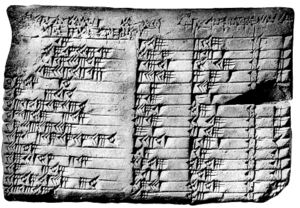There is debate whether the Pythagorean theorem was discovered once, or many times in many places.

The history of the theorem can be divided into four parts: knowledge of Pythagorean triples, knowledge of the relationship among the sides of a right triangle
Right triangle
A right triangle or right-angled triangle is a triangle in which one angle is a right angle . The relation between the sides and angles of a right triangle is the basis for trigonometry.-Terminology:The side opposite the right angle is called the hypotenuse...

, knowledge of the relationships among adjacent angles, and proofs of the theorem within some deductive system.

Bartel Leendert van der Waerden
Bartel Leendert van der Waerden
Bartel Leendert van der Waerden was a Dutch mathematician and historian of mathematics....

conjectured that Pythagorean triples were discovered algebra
Algebra
Algebra is the branch of mathematics concerning the study of the rules of operations and relations, and the constructions and concepts arising from them, including terms, polynomials, equations and algebraic structures...

ically by the Babylonians. Written between 2000 and 1786 BC, the Middle Kingdom
Middle Kingdom of Egypt
The Middle Kingdom of Egypt is the period in the history of ancient Egypt stretching from the establishment of the Eleventh Dynasty to the end of the Fourteenth Dynasty, between 2055 BC and 1650 BC, although some writers include the Thirteenth and Fourteenth dynasties in the Second Intermediate...

Egypt
Egypt
Egypt , officially the Arab Republic of Egypt, Arabic: , is a country mainly in North Africa, with the Sinai Peninsula forming a land bridge in Southwest Asia. Egypt is thus a transcontinental country, and a major power in Africa, the Mediterranean Basin, the Middle East and the Muslim world...

ian papyrus Berlin 6619
Berlin papyrus
The Berlin Papyrus 6619, commonly known as the Berlin Papyrus, is an ancient Egyptian papyrus document from the Middle Kingdom. This papyrus was found at the ancient burial ground of Saqqara in the early 19th century CE....

includes a problem whose solution is the Pythagorean triple
Pythagorean triple
A Pythagorean triple consists of three positive integers a, b, and c, such that . Such a triple is commonly written , and a well-known example is . If is a Pythagorean triple, then so is for any positive integer k. A primitive Pythagorean triple is one in which a, b and c are pairwise coprime...

6:8:10, but the problem does not mention a triangle. The Mesopotamia
Mesopotamia
Mesopotamia is a toponym for the area of the Tigris–Euphrates river system, largely corresponding to modern-day Iraq, northeastern Syria, southeastern Turkey and southwestern Iran.Widely considered to be the cradle of civilization, Bronze Age Mesopotamia included Sumer and the...

n tablet Plimpton 322
Plimpton 322
Plimpton 322 is a Babylonian clay tablet, notable as containing an example of Babylonian mathematics. It has number 322 in the G.A. Plimpton Collection at Columbia University...

, written between 1790
18th century BC
The 18th century BCE was the century which lasted from 1800 BCE to 1701 BCE.-Events:*1800 BCE: Iron age in India*1800 BCE: Beginning of the Nordic Bronze Age in the period system devised by Oscar Montelius....

and 1750 BC during the reign of Hammurabi
Hammurabi
Hammurabi Hammurabi Hammurabi (Akkadian from Amorite ʻAmmurāpi, "the kinsman is a healer", from ʻAmmu, "paternal kinsman", and Rāpi, "healer"; (died c...

the Great, contains many entries closely related to Pythagorean triples.

In India
India
India , officially the Republic of India , is a country in South Asia. It is the seventh-largest country by geographical area, the second-most populous country with over 1.2 billion people, and the most populous democracy in the world...

, the Baudhayana
Baudhayana
Baudhāyana, was an Indian mathematician, whowas most likely also a priest. He is noted as the author of the earliest Sulba Sūtra—appendices to the Vedas giving rules for the construction of altars—called the , which contained several important mathematical results. He is older than the other...

Sulba Sutra
Sulba Sutras
The Shulba Sutras or Śulbasūtras are sutra texts belonging to the Śrauta ritual and containing geometry related to fire-altar construction.- Purpose and origins :...

, the dates of which are given variously as between the 8th century BC and the 2nd century BC, contains a list of Pythagorean triples discovered algebraically, a statement of the Pythagorean theorem, and a geometrical
Geometry
Geometry arose as the field of knowledge dealing with spatial relationships. Geometry was one of the two fields of pre-modern mathematics, the other being the study of numbers ....

proof of the Pythagorean theorem for an isosceles right triangle.
The Apastamba
Apastamba
The Dharmasutra of Āpastamba forms a part of the larger Kalpasūtra of Āpastamba. It contains thirty praśnas, which literally means ‘questions’ or books. The subjects of this Dharmasūtra are well organized and preserved in good condition...

Sulba Sutra
(circa 600 BC) contains a numerical proof of the general Pythagorean theorem, using an area computation. Van der Waerden believes that "it was certainly based on earlier traditions". According to Albert Bŭrk, this is the original proof of the theorem; he further theorizes that Pythagoras visited Arakonam, India, and copied it. Boyer (1991) thinks the elements found in the Śulba-sũtram may be of Mesopotamian derivation.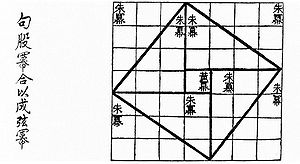With contents known much earlier, but in surviving texts dating from roughly the first century BC, the Chinese
China
Chinese civilization may refer to:* China for more general discussion of the country.* Chinese culture* Greater China, the transnational community of ethnic Chinese.* History of China* Sinosphere, the area historically affected by Chinese culture...

text Zhou Bi Suan Jing (周髀算经), (The Arithmetical Classic of the Gnomon and the Circular Paths of Heaven) gives an argument for the Pythagorean theorem for the (3, 4, 5) triangle—in China it is called the "Gougu Theorem" (勾股定理). During the Han Dynasty
Han Dynasty
The Han Dynasty was the second imperial dynasty of China, preceded by the Qin Dynasty and succeeded by the Three Kingdoms . It was founded by the rebel leader Liu Bang, known posthumously as Emperor Gaozu of Han. It was briefly interrupted by the Xin Dynasty of the former regent Wang Mang...

, from 202 BC to 220 AD, Pythagorean triples appear in The Nine Chapters on the Mathematical Art
The Nine Chapters on the Mathematical Art
The Nine Chapters on the Mathematical Art is a Chinese mathematics book, composed by several generations of scholars from the 10th–2nd century BCE, its latest stage being from the 1st century CE...

, together with a mention of right triangles. Some believe the theorem arose first in China
China
Chinese civilization may refer to:* China for more general discussion of the country.* Chinese culture* Greater China, the transnational community of ethnic Chinese.* History of China* Sinosphere, the area historically affected by Chinese culture...

, where it is alternatively known as the "Shang Gao Theorem" (商高定理), named after the Duke of Zhou's astrologer, and described in the mathematical collection Zhou Bi Suan Jing.

Pythagoras
Pythagoras
Pythagoras of Samos was an Ionian Greek philosopher, mathematician, and founder of the religious movement called Pythagoreanism. Most of the information about Pythagoras was written down centuries after he lived, so very little reliable information is known about him...

, whose dates are commonly given as 569–475 BC, used algebraic methods to construct Pythagorean triples, according to Proclus
Proclus
Proclus Lycaeus , called "The Successor" or "Diadochos" , was a Greek Neoplatonist philosopher, one of the last major Classical philosophers . He set forth one of the most elaborate and fully developed systems of Neoplatonism...

's commentary on Euclid
Euclid
Euclid , fl. 300 BC, also known as Euclid of Alexandria, was a Greek mathematician, often referred to as the "Father of Geometry". He was active in Alexandria during the reign of Ptolemy I...

. Proclus, however, wrote between 410 and 485 AD. According to Sir Thomas L. Heath
T. L. Heath
Sir Thomas Little Heath was a British civil servant, mathematician, classical scholar, historian of ancient Greek mathematics, translator, and mountaineer. He was educated at Clifton College...

, no specific attribution of the theorem to Pythagoras exists in the surviving Greek literature from the five centuries after Pythagoras lived. However, when authors such as Plutarch
Plutarch
Plutarch then named, on his becoming a Roman citizen, Lucius Mestrius Plutarchus , c. 46 – 120 AD, was a Greek historian, biographer, essayist, and Middle Platonist known primarily for his Parallel Lives and Moralia...

and Cicero
Cicero
Marcus Tullius Cicero , was a Roman philosopher, statesman, lawyer, political theorist, and Roman constitutionalist. He came from a wealthy municipal family of the equestrian order, and is widely considered one of Rome's greatest orators and prose stylists.He introduced the Romans to the chief...

attributed the theorem to Pythagoras, they did so in a way which suggests that the attribution was widely known and undoubted. "Whether this formula is rightly attributed to Pythagoras personally, [...] one can safely assume that it belongs to the very oldest period of Pythagorean mathematics."

Around 400 BC, according to Proclus, Plato
Plato
Plato , was a Classical Greek philosopher, mathematician, student of Socrates, writer of philosophical dialogues, and founder of the Academy in Athens, the first institution of higher learning in the Western world. Along with his mentor, Socrates, and his student, Aristotle, Plato helped to lay the...

gave a method for finding Pythagorean triples that combined algebra and geometry. Circa 300 BC, in Euclid's Elements
Euclid's Elements
Euclid's Elements is a mathematical and geometric treatise consisting of 13 books written by the Greek mathematician Euclid in Alexandria c. 300 BC. It is a collection of definitions, postulates , propositions , and mathematical proofs of the propositions...

, the oldest extant axiomatic proof
Mathematics
Mathematics is the study of quantity, space, structure, and change. Mathematicians seek out patterns and formulate new conjectures. Mathematicians resolve the truth or falsity of conjectures by mathematical proofs, which are arguments sufficient to convince other mathematicians of their validity...

of the theorem is presented.

## Pop references to the Pythagorean theorem

The Pythagorean theorem has arisen in popular culture
Popular culture
Popular culture is the totality of ideas, perspectives, attitudes, memes, images and other phenomena that are deemed preferred per an informal consensus within the mainstream of a given culture, especially Western culture of the early to mid 20th century and the emerging global mainstream of the...

in a variety of ways.
• A verse of the Major-General's Song
Major-General's Song
I Am the Very Model of a Modern Major-General is a patter song from Gilbert and Sullivan's 1879 comic opera The Pirates of Penzance. It is perhaps the most famous song in Gilbert and Sullivan's operas. It is sung by Major-General Stanley at his first entrance, towards the end of Act I...

in the Gilbert and Sullivan
Gilbert and Sullivan
Gilbert and Sullivan refers to the Victorian-era theatrical partnership of the librettist W. S. Gilbert and the composer Arthur Sullivan . The two men collaborated on fourteen comic operas between 1871 and 1896, of which H.M.S...

comic opera The Pirates of Penzance
The Pirates of Penzance
The Pirates of Penzance; or, The Slave of Duty is a comic opera in two acts, with music by Arthur Sullivan and libretto by W. S. Gilbert. The opera's official premiere was at the Fifth Avenue Theatre in New York City on 31 December 1879, where the show was well received by both audiences...

, "About binomial theorem I'm teeming with a lot o' news, With many cheerful facts about the square of the hypotenuse", makes an oblique reference to the theorem.
• The Scarecrow
Scarecrow (Oz)
The Scarecrow is a character in the fictional Land of Oz created by American author L. Frank Baum and illustrator William Wallace Denslow. In his first appearance, the Scarecrow reveals that he lacks a brain and desires above all else to have one. In reality, he is only two days old and merely...

in the film The Wizard of Oz
The Wizard of Oz (1939 film)
The Wizard of Oz is a 1939 American musical fantasy film produced by Metro-Goldwyn-Mayer. It was directed primarily by Victor Fleming. Noel Langley, Florence Ryerson and Edgar Allan Woolf received credit for the screenplay, but there were uncredited contributions by others. The lyrics for the songs...

makes a more specific reference to the theorem. Upon receiving his diploma from the Wizard
Wizard (Oz)
The Wizard of Oz, known during his reign as The Great and Powerful Oz, is the epithet of Oscar Zoroaster Phadrig Isaac Norman Henkel Emmannuel Ambroise Diggs, a fictional character in the Land of Oz, created by American author L...

, he immediately exhibits his "knowledge" by reciting a mangled and incorrect version of the theorem: "The sum of the square roots of any two sides of an isosceles triangle is equal to the square root of the remaining side. Oh, joy! Oh, rapture! I've got a brain!"
• In 2000, Uganda
Uganda
Uganda , officially the Republic of Uganda, is a landlocked country in East Africa. Uganda is also known as the "Pearl of Africa". It is bordered on the east by Kenya, on the north by South Sudan, on the west by the Democratic Republic of the Congo, on the southwest by Rwanda, and on the south by...

released a coin with the shape of an isosceles right triangle. The coin's tail has an image of Pythagoras and the equation α2 + β2 = γ2, accompanied with the mention "Pythagoras Millennium". Greece
Greece
Greece , officially the Hellenic Republic , and historically Hellas or the Republic of Greece in English, is a country in southeastern Europe....

, Japan
Japan
Japan is an island nation in East Asia. Located in the Pacific Ocean, it lies to the east of the Sea of Japan, China, North Korea, South Korea and Russia, stretching from the Sea of Okhotsk in the north to the East China Sea and Taiwan in the south...

, San Marino
San Marino
San Marino, officially the Republic of San Marino , is a state situated on the Italian Peninsula on the eastern side of the Apennine Mountains. It is an enclave surrounded by Italy. Its size is just over with an estimated population of over 30,000. Its capital is the City of San Marino...

, Sierra Leone
Sierra Leone
Sierra Leone , officially the Republic of Sierra Leone, is a country in West Africa. It is bordered by Guinea to the north and east, Liberia to the southeast, and the Atlantic Ocean to the west and southwest. Sierra Leone covers a total area of and has an estimated population between 5.4 and 6.4...

, and Suriname
Suriname
Suriname , officially the Republic of Suriname , is a country in northern South America. It borders French Guiana to the east, Guyana to the west, Brazil to the south, and on the north by the Atlantic Ocean. Suriname was a former colony of the British and of the Dutch, and was previously known as...

have issued postage stamps depicting Pythagoras and the Pythagorean theorem.
• In Neal Stephenson
Neal Stephenson
Neal Town Stephenson is an American writer known for his works of speculative fiction.Difficult to categorize, his novels have been variously referred to as science fiction, historical fiction, cyberpunk, and postcyberpunk...

's speculative fiction Anathem
Anathem
Anathem is a speculative fiction novel by Neal Stephenson, published in 2008. Major themes include the many-worlds interpretation of quantum mechanics and the philosophical debate between Platonic realism and formalism.-Plot summary:...

, the Pythagorean theorem is referred to as 'the Adrakhonic theorem'. A geometric proof of the theorem is displayed on the side of an alien ship to demonstrate the aliens' understanding of mathematics.

• Pythagorean Theorem (more than 70 proofs from cut-the-knot
Cut-the-knot
Cut-the-knot is a free, advertisement-funded educational website maintained by Alexander Bogomolny and devoted to popular exposition of many topics in mathematics. The site has won more than 20 awards from scientific and educational publications, including a Scientific American Web Award in 2003,...

)
• Interactive proof in Java
Java (programming language)
Java is a programming language originally developed by James Gosling at Sun Microsystems and released in 1995 as a core component of Sun Microsystems' Java platform. The language derives much of its syntax from C and C++ but has a simpler object model and fewer low-level facilities...

of The Pythagorean Theorem
• Another interactive proof in Java
Java (programming language)
Java is a programming language originally developed by James Gosling at Sun Microsystems and released in 1995 as a core component of Sun Microsystems' Java platform. The language derives much of its syntax from C and C++ but has a simpler object model and fewer low-level facilities...

of The Pythagorean Theorem
• Pythagorean theorem with interactive animation
• Animated, Non-Algebraic, and User-Paced Pythagorean Theorem
• History topic: Pythagoras's theorem in Babylonian mathematics In HTML with Java-based interactive figures.